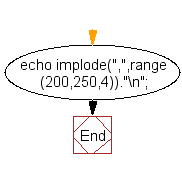﻿ PHP Array Exercise: Displays all the numbers between two numbers that are divisible by 4 - w3resource# PHP Array Exercises : Displays all the numbers between two numbers that are divisible by 4

## PHP Array: Exercise-13 with Solution

Write a PHP script which displays all the numbers between 200 and 250 that are divisible by 4.

Note : Do not use any PHP control statement.

Sample Solution:

PHP Code:

``````<?php
echo implode(",",range(200,250,4))."\n";
?>
```
```

Sample Output:

```200,204,208,212,216,220,224,228,232,236,240,244,248
```

Flowchart:PHP Code Editor:

What is the difficulty level of this exercise?

Test your Programming skills with w3resource's quiz.

﻿

## PHP: Tips of the Day

In PHP, there are two versions of logical AND and OR operators.

Operator True if
\$a and \$b Both \$a and \$b are true
\$a && \$b Both \$a and \$b are true
\$a or \$b Either \$a or \$b is true
\$a || \$b Either \$a or \$b is true

Note that the && and || opererators have higher precedence than and and or. See table below:

Evaluation Result of \$e Evaluated as
\$e = false || true True \$e = (false || true)
\$e = false or true False (\$e = false) or true

Because of this it's safer to use && and || instead of and and or.